# Momentum

Momentum
 Momentum SI symbol: p SI unit: kg · m/s Conserved: yes Derivations from other quantities: p = mv p = γm0v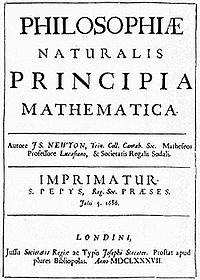Title page of the 1st edition of Isaac Newton's Principia defining the laws of motion.

In classical mechanics, linear momentum or translational momentum (pl. momenta; SI unit kg·m/s, or, equivalently, N·s) is the product of the mass and velocity of an object ($\mathbf{p} = m\mathbf{v}$). Like velocity, linear momentum is a vector quantity, possessing a direction as well as a magnitude.

Linear momentum is a conserved quantity, meaning that if a closed system is not affected by external forces, its total linear momentum cannot change. Although originally expressed in Newton's Second Law, the conservation of linear momentum also holds in special relativity and, with appropriate definitions, a (generalized) linear momentum conservation law holds in electrodynamics, quantum mechanics, quantum field theory, and general relativity. In relativistic mechanics, non-relativistic linear momentum is further multiplied by the Lorentz factor.

## History of the concept

Mōmentum was not merely the motion, which was mōtus, but was the power residing in a moving object, captured by today's mathematical definitions. A mōtus, "movement", was a stage in any sort of change, while velocitas, "swiftness", captured only speed. The concept of momentum in classical mechanics was originated by a number of great thinkers and experimentalists. The first of these was Byzantine philosopher John Philoponus, in his commentary to Aristotle´s Physics. As regards the natural motion of bodies falling through a medium, Aristotle's verdict that the speed is proportional to the weight of the moving bodies and indirectly proportional to the density of the medium is disproved by Philoponus through appeal to the same kind of experiment that Galileo was to carry out centuries later. This idea was refined by the European philosophers Peter Olivi and Jean Buridan. Buridan referred to impetus being proportional to the weight times the speed. Moreover, Buridan's theory was different to his predecessor's in that he did not consider impetus to be self dissipating, asserting that a body would be arrested by the forces of air resistance and gravity which might be opposing its impetus.

René Descartes believed that the total "quantity of motion" in the universe is conserved, where the quantity of motion is understood as the product of size and speed. This should not be read as a statement of the modern law of momentum, since he had no concept of mass as distinct from weight and size, and more importantly he believed that it is speed rather than velocity that is conserved. So for Descartes if a moving object were to bounce off a surface, changing its direction but not its speed, there would be no change in its quantity of motion. Galileo, later, in his Two New Sciences, used the Italian word "impeto".

The extent to which Isaac Newton contributed to the concept has been much debated. The answer is apparently nothing, except to state more fully and with better mathematics what was already known. Yet for scientists, this was the death knell for Aristotelian physics and supported other progressive scientific theories (i.e., Kepler's laws of planetary motion). Conceptually, the first and second of Newton's Laws of Motion had already been stated by John Wallis in his 1670 work, Mechanica sive De Motu, Tractatus Geometricus: "the initial state of the body, either of rest or of motion, will persist" and "If the force is greater than the resistance, motion will result". Wallis uses momentum and vis for force. Newton's Philosophiæ Naturalis Principia Mathematica, when it was first published in 1687, showed a similar casting around for words to use for the mathematical momentum. His Definition II defines quantitas motus, "quantity of motion", as "arising from the velocity and quantity of matter conjointly", which identifies it as momentum. Thus when in Law II he refers to mutatio motus, "change of motion", being proportional to the force impressed, he is generally taken to mean momentum and not motion. It remained only to assign a standard term to the quantity of motion. The first use of "momentum" in its proper mathematical sense is not clear but by the time of Jenning's Miscellanea in 1721, four years before the final edition of Newton's Principia Mathematica, momentum M or "quantity of motion" was being defined for students as "a rectangle", the product of Q and V, where Q is "quantity of material" and V is "velocity", s/t.

The Oxford English Dictionary dates the first print use of the word to 1699: John Keill, Reflections upon the Theory of the earth [written by himself] occasion'd by a late Examination of it, a letter (London).

Some languages, such as French still lack a single term for momentum, and use a phrase such as the literal translation of "quantity of motion". In Bulgarian and in Dutch the linear momentum is typically referred to as impulse, while the angular momentum is called momentum and the impulse has no distinct name.

## Linear momentum of a particle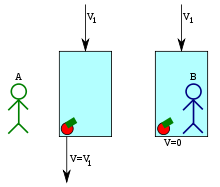Newton's apple in Einstein's elevator. In person A's frame of reference, the apple has non-zero velocity and momentum. In the elevator's and person B's frames of reference, it has zero velocity and momentum.

If an object is moving in any reference frame, then it has momentum in that frame. It is important to note that momentum is frame dependent. That is, the same object may have a certain momentum in one frame of reference, but a different amount in another frame. For example, a moving object has momentum in a reference frame fixed to a spot on the ground, while at the same time having 0 momentum in a reference frame attached to the object's center of mass.

The amount of momentum that an object has depends on two physical quantities: the mass and the velocity of the moving object in the frame of reference. In physics, the usual symbol for momentum is a bold p (bold because it is a vector); so this can be written$\mathbf{p}= m \mathbf{v}\,,$

where p is the momentum, m is the mass and v is the velocity.

Example: a model airplane of 1 kg traveling due north at 1 m/s in straight and level flight has a momentum of 1 kg·m/s due north measured from the ground. To the dummy pilot in the cockpit it has a velocity and momentum of zero.

According to Newton's second law, the rate of change of the momentum of a particle is proportional to the resultant force acting on the particle and is in the direction of that force. The derivation of force from momentum is given below.$\sum{\mathbf{F}} = {\mathrm{d}\mathbf{p} \over \mathrm{d}t} = m{\mathrm{d}\mathbf{v} \over \mathrm{d}t} + \mathbf{v}{\mathrm{d}m \over \mathrm{d}t}$

Given that mass is constant, the second term of the derivative is zero ($\mathbf{v}{\mathrm{d}m \over \mathrm{d}t} = 0$). We can therefore write the following:$\sum{\mathbf{F}} = m\mathbf{a}\,$

or just simply$\mathbf{F}= m \mathbf{a}\,,$

where F is understood to be the net force (or resultant).

Example: a model airplane of 1 kg accelerates from rest to a velocity of 1 m/s due north in 1 s. The thrust required to produce this acceleration is 1 newton. The change in momentum is 1 kg·m/s. To the dummy pilot in the cockpit there is no change of momentum. Its pressing backward in the seat is a reaction to the unbalanced thrust, shortly to be balanced by the drag.

## Linear momentum of a system of particles

### Relating to mass and velocity

The linear momentum of a system of particles is the vector sum of the momenta of all the individual objects in the system:$\mathbf{p}= \sum_{i = 1}^n m_i \mathbf{v}_i = m_1 \mathbf{v}_1 + m_2 \mathbf{v}_2 + m_3 \mathbf{v}_3 + \cdots + m_n \mathbf{v}_n\,,$

where p is the total momentum of the particle system, mi and vi are the respective mass and velocity of the i-th object, and n is the number of objects in the system.

It can be shown that, in the center of mass frame the momentum of a system is zero. Additionally, the momentum in a frame of reference that is moving at a velocity vcm with respect to that frame is simply:$\mathbf{p}= m\mathbf{v}_\text{cm}\,,$

where:$m=\sum_{i = 1}^n m_i\,.$

This is known as Euler's first law.

### Relating to force – General equations of motion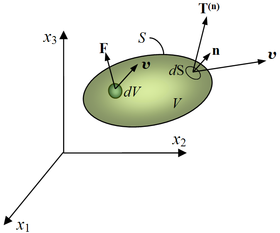Motion of a material body

The linear momentum of a system of particles can also be defined as the product of the total mass, m, of the system times the velocity, vcm, of the center of mass.$\sum{\mathbf{F}} = {\mathrm{d}\mathbf{p} \over \mathrm{d}t}= m \frac{\mathrm{d}\mathbf{v}_{cm}}{\mathrm{d}t}=m\mathbf{a}_{cm}\,$

This is a special case of Newton's second law (if mass is constant).

For a more general derivation using tensors, we consider a moving body (see Figure), assumed as a continuum, occupying a volume V, at a time t, having a surface area S, with defined traction or surface forces per unit area represented by the stress vector$T_i^{(n)}\,$ acting on every point of every body surface (external and internal), body forces Fi per unit of volume on every point within the volume V, and a velocity field vi, prescribed throughout the body. Following the previous equation, the linear momentum of the system is:$\int_S T_i^{(n)}dS + \int_V F_i dV = \frac{d}{dt}\int_V \rho\, v_i\, dV\,.$

By definition the stress vector is defined as$T_i^{(n)} \equiv \sigma_{ij}n_j\,$, then$\int_S \sigma_{ij}n_j\, dS + \int_V F_i\, dV = \frac{d}{dt}\int_V \rho\, v_i\, dV\,.$

Using the Gauss's divergence theorem to convert a surface integral to a volume integral gives (we denote$\partial_j \equiv \frac{\partial}{\partial x_j}\,$ as the differential operator):$\int_V \partial_j\sigma_{ij}\, dV + \int_V F_i\, dV = \frac{d}{dt}\int_V \rho\,v_i\, dV\,.$

Now we only need to take care of the right side of the equation. We have to be careful, since we cannot just take the differential operator under the integral. This is because while the motion of the continuum body is taking place (the body is not necessarily solid), the volume we are integrating on can change with time too. So the above integral will be:$\frac{d}{dt}\int \rho\,v_i\, dV=\int \frac{\partial (\rho v_i)}{\partial t}\, dV +\oint \rho v_i v_k n_k dA\,.$

Performing the differentiation in the first part, and applying the divergence theorem on the second part we obtain:$\frac{d}{dt}\int \rho\,v_i\, dV =\int \left[ \left(\rho\frac{\partial v_i}{\partial t}+v_i\frac{\partial \rho}{\partial t}\right)+\partial_k (\rho v_i v_k)\right]\, dV\,.$

Now the second term inside the integral is:$\partial_k (\rho v_i v_k)=\rho v_k \cdot \partial_k v_i +v_i\partial_k(\rho v_k)\,.$ Plugging this into the previous equation, and rearranging the terms, we get:$\frac{d}{dt}\int \rho\,v_i\, dV=\int\rho\left[\frac{\partial}{\partial t}+v_k\partial_k\right]v_i\,dV +\int\left[\frac{\partial\rho}{\partial t}+\partial_k(\rho v_k)\right]v_i\,dV\,.$

We can easily recognize the two integral terms in the above equation. The first integral contains the convective derivative of the velocity vector, and the second integral contains the change and flow of mass in time. Now lets assume that there are no sinks and sources in the system, that is mass is conserved, so this term is zero. Hence we obtain:$\frac{d}{dt}\int \rho\,v_i\, dV=\int \rho\,\frac{Dv_i}{Dt}\, dV\,$

putting this back into the original equation:$\int_V \left[ \partial_j\sigma_{ij} + F_i - \rho \frac{D v_i}{Dt}\right]\, dV = 0\,.$

For an arbitrary volume the integrand itself must be zero, and we have the Cauchy's equation of motion$\partial_j\sigma_{ij} + F_i = \rho \frac{D v_i}{Dt}\,.$

As we see the only extra assumption we made is that the system doesn't contain any mass sources or sinks, which means that mass is conserved. So this equation is valid for the motion of any continuum, even for that of fluids. If we are examining elastic continua only then the second term of the convective derivative operator can be neglected, and we are left with the usual time derivative, of the velocity field.

If a system is in equilibrium, the change in momentum with respect to time is equal to 0, as there is no acceleration$\sum{\mathbf{F}} = {\mathrm{d}\mathbf{p} \over \mathrm{d}t}=\ m\mathbf{a}_{cm}= 0\,$

or using tensors,$\partial_j\sigma_{ij} + F_i = 0\,.$

These are the equilibrium equations which are used in solid mechanics for solving problems of linear elasticity. In engineering notation, the equilibrium equations are expressed in Cartesian coordinates as$\frac{\partial \sigma_x}{\partial x} + \frac{\partial \tau_{yx}}{\partial y} + \frac{\partial \tau_{zx}}{\partial z} + F_x = 0\,$$\frac{\partial \tau_{xy}}{\partial x} + \frac{\partial \sigma_y}{\partial y} + \frac{\partial \tau_{zy}}{\partial z} + F_y = 0\,$$\frac{\partial \tau_{xz}}{\partial x} + \frac{\partial \tau_{yz}}{\partial y} + \frac{\partial \sigma_z}{\partial z} + F_z = 0\,.$

## Conservation of linear momentum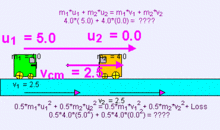This example (m1 = 4kg, u1 = 5m/s, m2 = 4kg, u2=0m/s) of collision carts in perfectly inelastic collision, notice the center of mass of the masses m1 and m2 is at the location of vcm = 2.5m/s always throughout the motion.

The law of conservation of linear momentum is a fundamental law of nature, and it states that if no external force acts on a closed system of objects, the momentum of the closed system remains constant. One of the consequences of this is that the center of mass of any system of objects will always continue with the same velocity unless acted on by a force from outside the system.

Conservation of momentum is a mathematical consequence of the homogeneity (shift symmetry) of space (position in space is the canonical conjugate quantity to momentum). That is, conservation of momentum is equivalent to the fact that the physical laws do not depend on position.

In analytical mechanics the conservation of momentum is a consequence of translational invariance of Lagrangian in the absence of external forces. It can be proven that the total momentum is a constant of motion by making an infinitesimal translation of Lagrangian and then equating it with non translated Lagrangian. This is a special case of Noether's theorem .

In an isolated system (one where external forces are absent) the total momentum will be constant: this is implied by Newton's first law of motion. Newton's third law of motion, the law of reciprocal actions, which dictates that the forces acting between systems are equal in magnitude, but opposite in sign, is due to the conservation of momentum.

Since position in space is a vector quantity, momentum (being the canonical conjugate of position) is a vector quantity as well—it has direction. Thus, when a gun is fired, the final total momentum of the system (the gun and the bullet) is the vector sum of the momenta of these two objects. Assuming that the gun and bullet were at rest prior to firing (meaning the initial momentum of the system was zero), the final total momentum must also equal 0.

In an isolated system with only two objects, the change in momentum of one object must be equal and opposite to the change in momentum of the other object. Mathematically,$\Delta \mathbf{p}_1 = -\Delta \mathbf{p}_2\,.$

Momentum has the special property that, in a closed system, it is always conserved, even in collisions and separations caused by explosive forces. Kinetic energy, on the other hand, is not conserved in collisions if they are inelastic. Since momentum is conserved it can be used to calculate an unknown velocity following a collision or a separation if all the other masses and velocities are known.

A common problem in physics that requires the use of this fact is the collision of two particles. Since momentum is always conserved, the sum of the momenta before the collision must equal the sum of the momenta after the collision:$m_1 \mathbf u_{1} + m_2 \mathbf u_{2} = m_1 \mathbf v_{1} + m_2 \mathbf v_{2}\,,$

where u1 and u2 are the velocities before collision, and v1 and v2 are the velocities after collision.

Determining the final velocities from the initial velocities (and vice versa) depend on the type of collision. There are two types of collisions that conserve momentum: elastic collisions, which also conserve kinetic energy, and inelastic collisions, which do not.

### Elastic collisions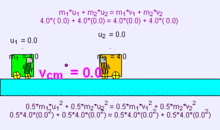Example (m1 = 4kg, u1 = 5m/s, m2 = 4kg, u2=0m/s) of collision carts in perfectly elastic collision showing the equations of momentum and kinetic energy both conserved

A collision between two pool balls is a good example of an almost totally elastic collision, due to their high rigidity; a totally elastic collision exists only in theory, occurring between bodies with mathematically infinite rigidity. In addition to momentum being conserved when the two balls collide, the sum of kinetic energy before a collision must equal the sum of kinetic energy after:$\tfrac{1}{2} m_1 u_{1}^2 + \tfrac{1}{2} m_2 u_{2}^2 = \tfrac{1}{2} m_1 v_{1}^2 + \tfrac{1}{2} m_2 v_{2}^2\,.$

#### In one dimension

When the initial velocities are known, the final velocities for a head-on collision are given by$\mathbf{v}_{1} = \left( \frac{m_1 - m_2}{m_1 + m_2} \right) \mathbf{u}_{1} + \left( \frac{2 m_2}{m_1 + m_2} \right) \mathbf{u}_{2}\,$$\mathbf{v}_{2} = \left( \frac{m_2 - m_1}{m_1 + m_2} \right) \mathbf{u}_{2} + \left( \frac{2 m_1}{m_1 + m_2} \right) \mathbf{u}_{1}\,.$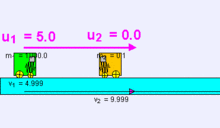This example m1=1000kg, u1=5m/s, m2=0.1kg, u2=0m/s, the final velocities are approximately given v1=4.999m/s, v2=9.999m/s

When the first body is much more massive than the other (that is, m1 » m2), the final velocities are approximately given by$\mathbf{v}_{1} = \mathbf{u}_{1}\,$$\mathbf{v}_{2} = 2\mathbf{u}_{1} - \mathbf{u}_{2}\,.$

Thus the more massive body does not change its velocity, and the less massive body travels at twice the velocity of the more massive body less its own original velocity. Assuming both masses were heading towards each other on impact, the less massive body is now therefore moving in the opposite direction at twice the speed of the more massive body plus its own original speed.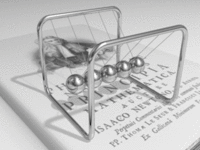A Newton's cradle demonstrates conservation of momentum.

In a head-on collision between two bodies of equal mass (that is, m1 = m2), the final velocities are given by$\mathbf{v}_1 = \mathbf{u}_2\,$$\mathbf{v}_2 = \mathbf{u}_1\,.$

Thus the bodies simply exchange velocities. If the first body has nonzero initial velocity u1 and the second body is at rest, then after collision the first body will be at rest and the second body will travel with velocity u1. This phenomenon is demonstrated by Newton's cradle.

#### In multiple dimensions

In the case of objects colliding in more than one dimension, as in oblique collisions, the velocity is resolved into orthogonal components with one component perpendicular to the plane of collision and the other component or components in the plane of collision. The velocity components in the plane of collision remain unchanged, while the velocity perpendicular to the plane of collision is calculated in the same way as the one-dimensional case.

For example, in a two-dimensional collision, the momenta can be resolved into x and y components. We can then calculate each component separately, and combine them to produce a vector result. The magnitude of this vector is the final momentum of the isolated system.

#### Perfectly inelastic collisionsExample (m1 = 4kg, u1 = 5m/s, m2 = 4kg, u2=0m/s) of collision carts in perfectly inelastic collision showing the equations of momentum conserved and kinetic energy associated with some energy loss.

A common example of a perfectly inelastic collision is when two snowballs collide and then stick together afterwards. This equation describes the conservation of momentum:$m_1 \mathbf u_{1} + m_2 \mathbf u_{2} = \left( m_1 + m_2 \right) \mathbf v\,$

It can be shown that a perfectly inelastic collision is one in which the maximum amount of kinetic energy is converted into other forms. For instance, if both objects stick together after the collision and move with a final common velocity, one can always find a reference frame in which the objects are brought to rest by the collision and 100% of the kinetic energy is converted. This is true even in the relativistic case and utilized in particle accelerators to efficiently convert kinetic energy into new forms of mass-energy (i.e. to create massive particles).

#### Coefficient of restitution

The coefficient of restitution is defined as the ratio of relative velocity of separation to relative velocity of approach. It is a ratio hence it is a dimensionless quantity. The coefficient of restitution is given by:$C_R = \frac{v_2 - v_1}{u_1 - u_2}$

for two colliding objects, where

v1 is the scalar final velocity of the first object after impact,
v2 is the scalar final velocity of the second object after impact,
u1 is the scalar initial velocity of the first object before impact,
u2 is the scalar initial velocity of the second object before impact.

A perfectly elastic collision implies that CR is 1. So the relative velocity of approach is same as the relative velocity of separation of the colliding bodies.

Inelastic collisions have (CR < 1). In case of a perfectly inelastic collision the relative velocity of separation of the centre of masses of the colliding bodies is 0. Hence the bodies stick together after collision.

#### Explosions

An explosion occurs as a result of a chain reaction that transforms potential energy into kinetic energy displacing the surrounding material. Explosions do not conserve potential energy. Instead, potential energy stored in chemical, mechanical, or nuclear form, is transformed into kinetic energy, acoustic energy, and electromagnetic radiation.

See the inelastic collision page for more details.

## Modern definitions of momentum

### Momentum in relativistic mechanics

In relativistic mechanics, in order to be conserved, the momentum of an object must be defined as$\mathbf{p} = \gamma m_0\mathbf{v}\,,$

where m0 is the invariant mass of the object and γ is the Lorentz factor, given by$\gamma = \frac{1}{\sqrt{1 - (v/c)^2}}\,,$

where v is the speed of the object and c is the speed of light. The inverse relation is given by:$\mathbf{v} = \frac{c^2 \mathbf{p}}{\sqrt{(pc)^2 + (m_0 c^2)^2}} = \frac{c^2 \mathbf{p}}{E} \,,$

where$p = \sqrt{p_x^2 + p_y^2 + p_z^2}$ is the magnitude of the momentum.

Relativistic momentum can also be written as invariant mass times the object's proper velocity, defined as the rate of change of object position in the observer frame with respect to time elapsed on object clocks (i.e. object proper time). Within the domain of classical mechanics, relativistic momentum closely approximates Newtonian momentum: at low velocity, γm0v is approximately equal to m0v, the Newtonian expression for momentum.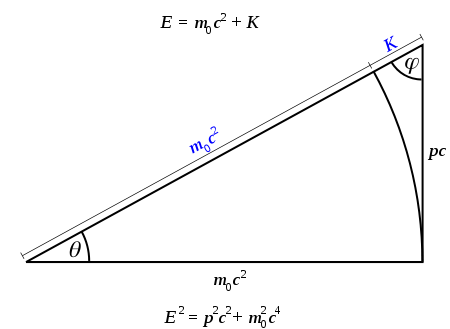A graphical representation of the interrelation of relativistic energy E, invariant mass m0, relativistic momentum p, and relativistic mass m = γm0.

The total energy E of a body is related to the relativistic momentum p by$E^2 = (pc)^2 + (m_0c^2)^2\,,$

where p denotes the magnitude of p. This relativistic energy-momentum relationship holds even for massless particles such as photons; by setting m0 = 0 it follows that$E = pc\,.$

For both massive and massless objects, relativistic momentum is related to the de Broglie wavelength λ by$p = h/\lambda\,,$

where h is the Planck constant.

#### Four-vector formulation

Relativistic four-momentum as proposed by Albert Einstein arises from the invariance of four-vectors under Lorentzian translation. The four-momentum P is defined as:$\mathbf{P} := (E/c, p_x , p_y ,p_z)\,,$

where E = γm0c2 is the total relativistic energy of the system, and px, py, and pz represent the x-, y-, and z-components of the relativistic momentum, respectively.

The magnitude ||P|| of the momentum four-vector is equal to m0c, since$||\mathbf{P}||^2 = (E/c)^2 - p^2 = (m_0c)^2\,.$

which is invariant across all reference frames. For a closed system, the total four-momentum is conserved, which effectively combines the conservation of both momentum and energy into a single equation. For example, in the radiationless collision of two particles with rest masses m1 and m2 with initial velocities$\mathbf{v}_1$ and$\mathbf{v}_2$, the respective final velocities$\mathbf{v}_3$ and$\mathbf{v}_4$ may be found from the conservation of four-momentum which states that:$\mathbf{P}_1+\mathbf{P}_2=\mathbf{P}_3+\mathbf{P}_4\,,$

where$\mathbf{P}_i=m_i\gamma_i(c,\mathbf{v}_i).$

For elastic collisions, the rest masses remain the same (m1 = m3 and m2 = m4), while for inelastic collisions, the rest masses will increase after collision due to an increase in their heat energy content. The conservation of four-momentum can be shown to be the result of the homogeneity of space–time.

#### Generalization of momentum

Momentum is the Noether charge of translational invariance. As such, not just particles, but fields and other things can have momentum. However, where space–time is curved there is no Noether charge for translational invariance.

### Momentum in quantum mechanics

In quantum mechanics, momentum is defined as an operator on the wave function. The Heisenberg uncertainty principle defines limits on how accurately the momentum and position of a single observable system can be known at once. In quantum mechanics, position and momentum are conjugate variables.

For a single particle described in the position basis the momentum operator can be written as$\mathbf{p}={\hbar\over i}\nabla=-i\hbar\nabla\,,$

where ∇ is the gradient operator, ħ is the reduced Planck constant, and i is the imaginary unit. This is a commonly encountered form of the momentum operator, though the momentum operator in other bases can take other forms, for example in the momentum basis the momentum operator is represented as$\mathbf{p}\psi(p) = p\psi(p)\,,$

where the operator p acting on a wave function ψ(p) yields that wave function multiplied by the value p, in an analogous fashion to the way that the position operator acting on a wave function ψ(x) yields that wave function multiplied by the value x.

### Momentum in electromagnetism

Electric and magnetic fields possess momentum regardless of whether they are static or they change in time. The pressure, P, of an electrostatic (magnetostatic) field upon a metal sphere, cylindrical capacitor or ferromagnetic bar is:$P_{static} = {W}= \left[ {\epsilon_0 \epsilon}{\frac{{\mathbf E}^2 }{ {2}}} +{\frac{ 1 }{ {\mu_0 \mu} }} {\frac{{\mathbf B}^2}{{2}}} \right]\,,$

where${W}\,$,${\mathbf E}\,$,${\mathbf B}\,$, are the electromagnetic energy density, electric field, and magnetic field respectively. The electromagnetic pressure${P}={W}\,$ may be sufficiently high to explode the capacitor. Thus electric and magnetic fields do carry momentum.

Light (visible, UV, radio) is an electromagnetic wave and also has momentum. Even though photons (the particle aspect of light) have no mass, they still carry momentum. This leads to applications such as the solar sail. The calculation of the momentum of light within dielectric media is somewhat controversial (see Abraham–Minkowski controversy ).

Momentum is conserved in an electrodynamic system (it may change from momentum in the fields to mechanical momentum of moving parts). The treatment of the momentum of a field is usually accomplished by considering the so-called energy-momentum tensor and the change in time of the Poynting vector integrated over some volume. This is a tensor field which has components related to the energy density and the momentum density.

The definition of canonical momentum corresponding to the momentum operator of quantum mechanics when it interacts with the electromagnetic field is, using the principle of least coupling:$\mathbf P = m\mathbf v + q\mathbf A\,$,$\mathbf p = m\mathbf v\,,$

where:$\mathbf A\,$ is the electromagnetic vector potential$m\,$ the charged particle's invariant mass$\mathbf v\,$ its velocity$q\,$ its charge.

## Analogies between heat, mass, and momentum transfer

There are some notable similarities in equations for momentum, heat, and mass transfer. The molecular transfer equations of Newton's law for fluid momentum, Fourier's law for heat, and Fick's law for mass are very similar. A great deal of effort has been devoted to developing analogies among these three transport processes so as to allow prediction of one from any of the others.

Wikimedia Foundation. 2010.

Synonyms:

### Look at other dictionaries:

• Momentum — (sächlich, lateinisch mōmentum, „(Dauer einer) Bewegung“) steht für: einen Film aus dem Jahre 2003, siehe Momentum (Film) ein Verfahren aus der Chartanalyse, siehe Momentum (Chartanalyse) die Investmentgesellschaft Momentum, die im Jahr 2002 von… …   Deutsch Wikipedia

• Momentum — Mo*men tum, n.; pl. L. {Momenta}, F. {Momentums}. [L. See {Moment}.] 1. (Mech.) The quantity of motion in a moving body, being always proportioned to the quantity of matter multiplied by the velocity; impetus. [1913 Webster] 2. Essential element …   The Collaborative International Dictionary of English

• momentum — (n.) 1690s, scientific use in mechanics, quantity of motion of a moving body, from L. momentum movement, moving power (see MOMENT (Cf. moment)). Figurative use dates from 1782 …   Etymology dictionary

• momentum — [mō men′təm, məmen′təm] n. pl. momentums or momenta [mō men′tə] [ModL < L: see MOMENT] 1. the impetus of a moving object 2. strength or force that keeps growing [a campaign that gained momentum] 3. Physics Mech. the product of the mass of a… …   English World dictionary

• momentum — I index headway, impetus II index importance, stress (strain) Burton s Legal Thesaurus …   Law dictionary

• momentum — impetus, *speed, velocity, pace, headway …   New Dictionary of Synonyms

• momentum — The plural, though not often needed, is momenta, or informally momentums …   Modern English usage

• momentum — [n] impetus, push drive, energy, force, impulse, power, propulsion, strength, thrust; concepts 641,712 …   New thesaurus

• momentum — ► NOUN (pl. momenta) 1) impetus gained by movement or progress. 2) Physics the quantity of motion of a moving body, equal to the product of its mass and velocity. ORIGIN Latin movimentum, from movere to move …   English terms dictionary

• momentum — /moh men teuhm/, n., pl. momenta / teuh/, momentums. 1. force or speed of movement; impetus, as of a physical object or course of events: The car gained momentum going downhill. Her career lost momentum after two unsuccessful films. 2. Mech. a… …   Universalium